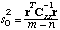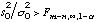# Manual Page for Command >>> FISHER

Syntax
>>> FISHER

Parent Command
>> ERROR

Subcommand
-

Description
The estimated error variance s02 represents the variance of the mean weighted residual and is thus a measure of goodness-of-fit:The value s02 is used in the subsequent error analysis. For example, the covariance matrix of the estimated parameters, Cpp, is directly proportional to the scalar s02. Note that if the residuals are consistent with the distributional assumption about the measurement errors (i.e., matrix Czz), then the estimated error variance assumes a value close to one. s02 is also an estimate for the true or a priori error variance sigma02. It can be shown that the ratio (s02/sigma02) follows an F-distribution with the two degrees of freedom f1=m-n, and f2=infinity. Therefore, it can be statistically tested to see whether the final match deviates significantly from the modeler’s expectations, expressed by matrix Czz. This is called the Fisher Model Test. The user must decide whether the error analysis should be based on the a posteriori or a priori error variance (see commands >>> POSTERIORI and >>> PRIORI, respectively). The decision can also be delegated to the Fisher Model Test according to the following table:

Fisher Model Test Error Variance Commenterror either in the functional or stochastic modelmodel test passedprobably error in stochastic model

Example
> COMPUTATION
>> ERROR
>>> let the FISHER model test decide whether the
a priori or a posteriori error variance should be used
>>> confidence level 1-ALPHA : 95 %
<<<